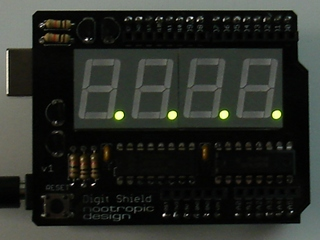Select Page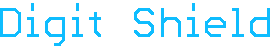Easily add a four digit 7-segment display to your Arduino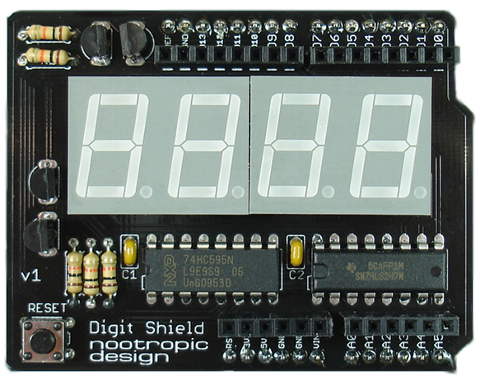The Digit Shield is a simple, low-cost Arduino shield that provides a four digit 7-segment display.

• An easy-to-use open source library makes it very simple to display integers and floating point numbers.
• Multiplexing of digits is implemented using a timer overflow interrupt handler for flicker-free display.
• Only uses 4 Arduino pins (2,3,4,5).
• All through-hole parts for easy kit assembly.
• Available in three display colors: fiery red, crisp green, or ice blue!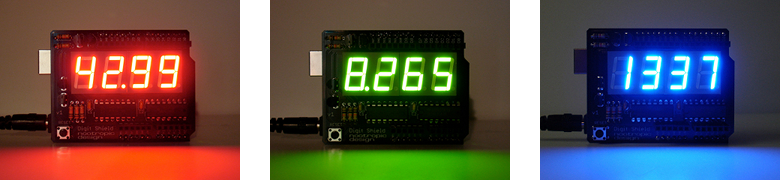## Digit Shield Library

Using the Digit Shield is extremely simple when you use the Digit Shield library. Download the library and install it in your Arduino libraries folder just like any other library. There are many examples that demonstrate different features of the API.

Here’s a summary of the library API:

DigitShield.begin() : initialize the Digit Shield. This is required before use.
DigitShield.setValue(int value) : set an integer value to display
DigitShield.setValue(double value) : set a floating point value to display
DigitShield.setPrecision(int decimalPlaces) : specify the number of decimal places to display
DigitShield.setLeadingZeros(boolean b) : specify whether numbers should be displayed with leading zeros (default is false)
DigitShield.setBlank(boolean b) : allows you to blank the display completely. Specify false to turn the display back on.
DigitShield.setDigit(int d, int n) : set digit d to value n. Digits are numbered 1-4 from left to right.
DigitShield.setDecimalPoint(int d, boolean on) : turn the decimal point of digit d on or off.

## Examples

Display the value 123:

`DigitShield.setValue(123);`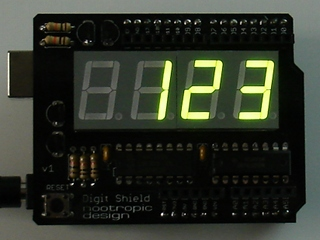Display the value 64 with leading zeros:

```DigitShield.setLeadingZeros(true);
DigitShield.setValue(64);```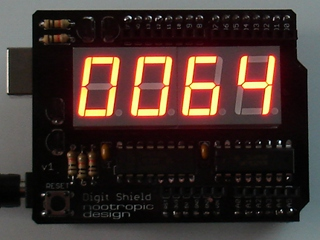Display the value of pi with 2 decimal places

```DigitShield.setPrecision(2);
DigitShield.setValue(3.14159265);```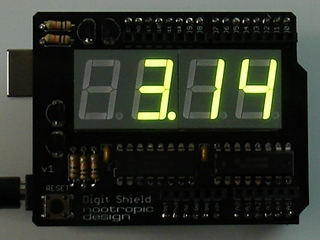Display the value 0.012. Floating point values less than 1.0 will have a zero before the decimal point.

```DigitShield.setPrecision(3);
DigitShield.setValue(.012);```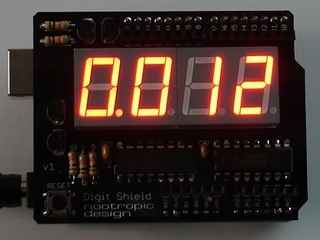Display the value 43 with leading zeros and one decimal place.

```DigitShield.setLeadingZeros(true);
DigitShield.setPrecision(1);
DigitShield.setValue(43);```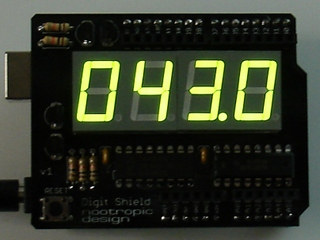Access the digits and decimal points directly. Digits are numbered 1-4 from left to right. For example, to display the score of a game.

```DigitShield.setDigit(1, 7);
DigitShield.setDigit(4, 3);
DigitShield.setDecimalPoint(2, true);```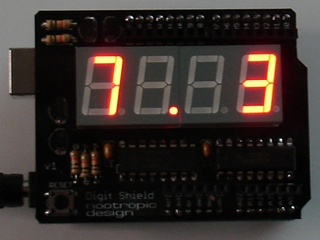Error conditions: if the value cannot be displayed because it will not fit on the display, this error is indicated by lighting all 4 decimal points. The following cases are all error conditions:

```DigitShield.setValue(10000);  // too large
```
```DigitShield.setPrecision(3);
DigitShield.setValue(83.1415);  // not enough room
```
`DigitShield.setValue(-32);  // no negative numbers`